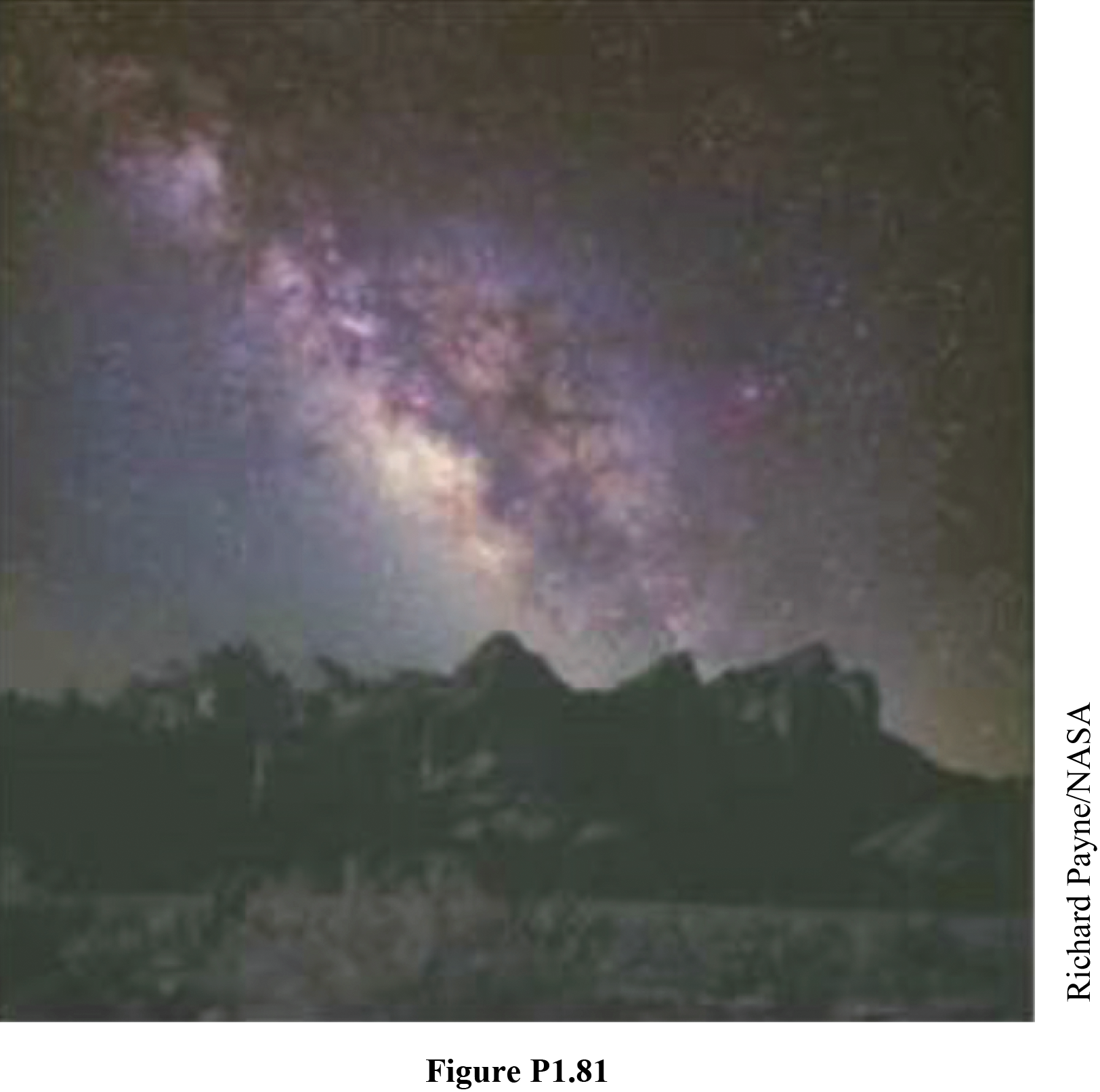Chapter 1, Problem 81AP

Chapter
Section
Textbook Problem

The nearest neutron star (a collated star made primarily of neutrons) is about 3.00 × 1018 m away from Earth. Given that the Milky Way galaxy (Fig. P1.81) is roughly a disk of diameter ~ 1021 m and thickness ~ 1019 m, estimate the number of neutron stars in the Milky Way to the nearest order of magnitude.Figure P1.81

To determine
The number of neutron star in the Milky Way to the nearest order of magnitude.

Explanation

Given Info: The nearest neutron star from Earth is 3×1018m away. The diameter of Milky Way galaxy is 1021m and thickness is 1019m .

Explanation:

Formula to calculate the volume of the galaxy is,

Vg=π(d24)t

• Vg is volume of the galaxy,
• d is diameter of the galaxy,
• t is the thickness of the galaxy

Substitute 1021m for d , 1019m for t to find Vg .

Vg=π((1021m)24)(1019m)1061m3

Formula to calculate the galactic volume of the neutron star is,

V1=4

Still sussing out bartleby?

Check out a sample textbook solution.

See a sample solution

The Solution to Your Study Problems

Bartleby provides explanations to thousands of textbook problems written by our experts, many with advanced degrees!

Get Started